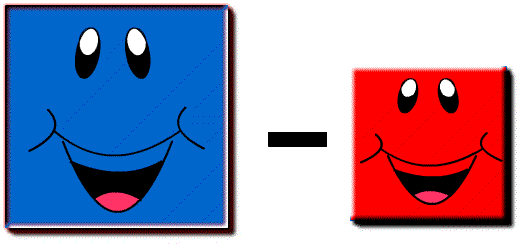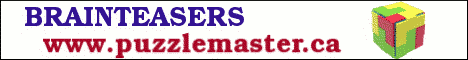#Write down two consecutive numbers.

Square each of them and find the difference.

Do the same for other pairs of consecutive numbers.

What do you notice?## A Mathematics Lesson Starter Of The Day

Share

Topics: Starter | Number

• Mr Frost, John Summers High School
•
• The difference of the squares of two consequetive numbers will always equal the sum of those two numbers.

sum of the numbers:
a + (a-1) = 2a - 1

Difference of the square of the numbers
a2 - (a-1)2 = a2 - (a2 - 2a + 1)
= 2a - 1
• Mr Frost, John Summers High School, Flintshire
•
• or

Difference in squares
a2 - (a + 1)2 = a2 - (a2 + 2a + 1)
= 2a + 1

Sum of the numbers

a + (a + 1) = 2a + 1
• David Longman, Bedfordshire Middle School
•
• As an extension of this idea

a² - b² = (a + b) x (a - b) wherever a is greater than b
• Steve Eastop, Margate, Kent
•
• The difference between the results of squaring each consecutive number and then subtracting the lesser result from the greater result always results in an ODD INTEGER (i.e. a positive or negative whole number indivisible by two). In other words, the result to such a calculation will always be a member of the set {...-5,-3,-1,1,3,5,7,9,11,....}.
In general, algebraically, let the two consecutive numbers be: (N-1) and (N) respectively.(whereby N is the larger of the two).
Then (N)^2 - (N-1)^2 = (N^2) - ((N-1)(N-1)) (expanding and simplifying)
= N^2 - (N^2 - N - N + 1)
= N^2 - N^2 + N + N + 1
= (2N +1).
Hence, whatever integral value of N you assign, 2N will always be even and thus (2N + 1) will be odd as already stated above!

How did you use this starter? Can you suggest how teachers could present or develop this resource? Do you have any comments? It is always useful to receive feedback and helps make this free resource even more useful for Maths teachers anywhere in the world.
Click here to enter your comments.

If you don't have the time to provide feedback we'd really appreciate it if you could give this page a score! We are constantly improving and adding to these starters so it would be really helpful to know which ones are most useful. Simply click on a button below:

Excellent, I would like to see more like this
Good, achieved the results I required
Satisfactory
Didn't really capture the interest of the students
Not for me! I wouldn't use this type of activity.

This starter has scored a mean of 3.0 out of 5 based on 384 votes.

Previous Day | This starter is for 10 October | Next Day

## Answers

 22 - 12 = 3 32 - 22 = 5 42 - 32 = 7 52 - 42 = 9 62 - 52 = 11 72 - 62 = 13 82 - 72 = 15 92 - 82 = 17 102 - 92 = 19 112 - 102 = 21 122 - 112 = 23 132 - 122 = 25 142 - 132 = 27 152 - 142 = 29 162 - 152 = 31 172 - 162 = 33 182 - 172 = 35 192 - 182 = 37 202 - 192 = 39 212 - 202 = 41 222 - 212 = 43 232 - 222 = 45 242 - 232 = 47 252 - 242 = 49 262 - 252 = 51 272 - 262 = 53 282 - 272 = 55 292 - 282 = 57 302 - 292 = 59 312 - 302 = 61 322 - 312 = 63 332 - 322 = 65 342 - 332 = 67 352 - 342 = 69 362 - 352 = 71 372 - 362 = 73 382 - 372 = 75 392 - 382 = 77 402 - 392 = 79 412 - 402 = 81 422 - 412 = 83 432 - 422 = 85 442 - 432 = 87 452 - 442 = 89 462 - 452 = 91 472 - 462 = 93 482 - 472 = 95 492 - 482 = 97 502 - 492 = 99 512 - 502 = 101 522 - 512 = 103

Your access to the majority of the Transum resources continues to be free but you can help support the continued growth of the website by doing your Amazon shopping using the links on this page. Below is an Amazon search box and some items chosen and recommended by Transum Mathematics to get you started.Teacher, do your students have access to computers?Do they have iPads or Laptops in Lessons? Whether your students each have a TabletPC, a Surface or a Mac, this activity lends itself to eLearning (Engaged Learning).Here a concise URL for a version of this page without the comments.

Transum.org/go/?Start=October10

Here is the URL which will take them to another activity involving square numbers.

Transum.org/go/?to=sqpgFor All:

©1997-2019 WWW.TRANSUM.ORG